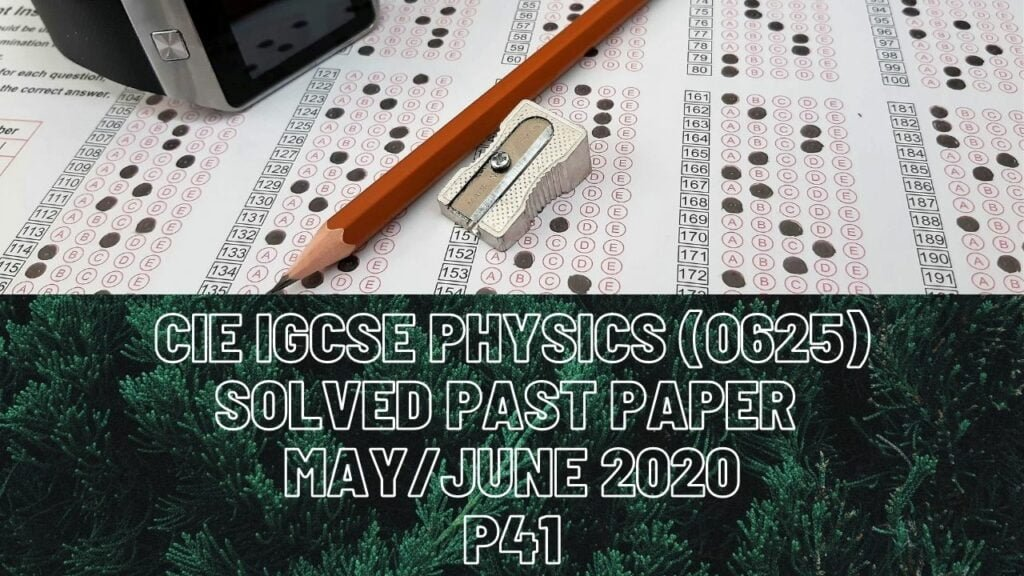#### CIE IGCSE Physics Solved Past Paper May/June 2020 P41

2a) From 0 to 8 b) Force = constant*extensionConstant value = force/extension= 8/15= 0.53 N/cmci) Elastic potential energyii) Extension = force/constant= (4/0.54)+(4/0.54)= 14.8 =15 cm of extensioniii) So now extension is 6 instead of four here the increase in extension is6-4=2. 2 is half of 4 so if new extension is half of the earlier extension,New distance should also be…